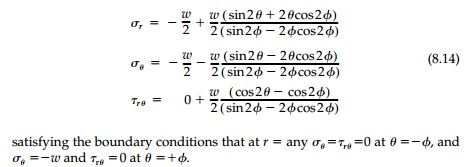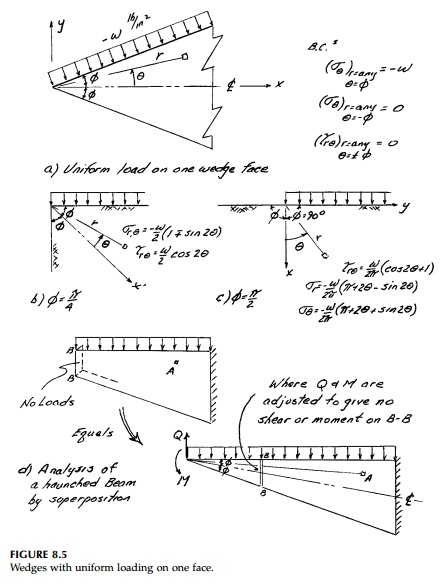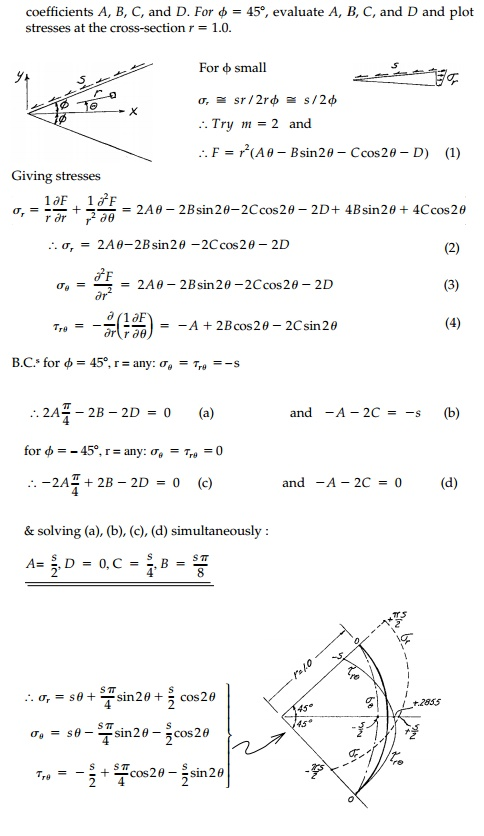## Chapter: Civil : Principles of Solid Mechanics : Wedges and the Half-Space

A similar paradox and a clue to its source and possible resolution can be seen clearly in the solution for a uniform (isotropic) loading on each face (Figure 8.4a) of a wedge.

A similar paradox and a clue to its source and possible resolution can be seen clearly in the solution for a uniform (isotropic) loading on each face (Figure 8.4a) of a wedge. From the boundary condition that at any radius when,m must equal 2 and using only the symmetric termsClearly, since there is no singularity in the solution, its nonexistence for 2ϕ = 180o introduces a more fundamental ambiguity. For wedge angles less than 90o where there is no constraint on Eθ no matter what the radius, the isotropic field seems reasonable, but for 2ϕ > 90o , it seems suspect. Certainly equilib-rium, compatibility, and the stress boundary conditions are satisfied for any wedge angle, but there seems to be some added condition on deformations that precludes a simple isotropic field.

The same is true for the accepted solution for a wedge with uniform tension and compression on the faces (Figure 8.4b). Again, by similar reasoning, m = 2, the distribution of σr should be asymmetric and, therefore, we choose:Again this solution cannot be correct in the far field for the half-space where the horizontal stress σy is a function of v. Moreover, the solution has the same singularity at 2ϕ= 257.4o as in the case of a line moment at the apex [Equation (8.8)]. Again the denominator sin2ϕ = 2ϕcos2ϕ goes to zero and the solution blows up.

Finally, if we add the two previous cases together as in Figure 8.4 where w/2=q, this gives the solution for a wedge with a uniform load along the top surface (Figure 8.5).The special cases of a vertical cut, ϕ=45o , and the half-space are shown in Figure 8.5 b and c. Again in the far field under the load, the solution is sus-pect, but near the origin it gives sensible results. An example of how wedge solutions can be combined to give a solution for a haunched beam with uni-form loading is shown in Figure 8.5d. Other examples are given in the chap-ter problems.*Example 8.2

A wedge is loaded by a uniform shearing traction, s, on its “upper” face as shown below. Determine the general stress function in terms of unknown coefficients A, B, C, and D.Study Material, Lecturing Notes, Assignment, Reference, Wiki description explanation, brief detail
Civil : Principles of Solid Mechanics : Wedges and the Half-Space : Uniform Loading Cases |

Related Topics